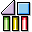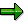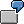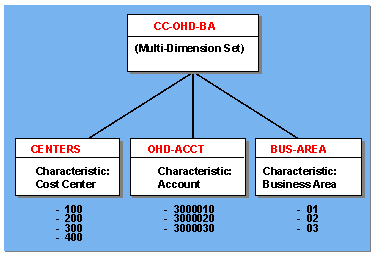VariablesPurpose

A variable is a placeholder for data to be specified when you run a report that uses the variable. Variables are used in many areas, for example in reporting ( Report Painter / Report Writer). For example, a variable could be a company, the current year, the current month, a date, or a user name.When you use a variable in a report, the value that you choose for the variable is valid for only one report run. Each time you run a report, you can enter a new value for the variables used in the report.

Features

You can create the following types of variables:

• Value variables

This variable represents a specific value that you can enter directly. You can only use value variables in basic sets. For more information, see entering value and formula variables in a set.You can create a variable called YEAR. When you enter the variable YEAR in a basic set, and then you use the basic set in a report, the system prompts you to enter a year when you run the report.

• Formula variables

This variable represents a formula, which determines a value for the variable. You can only use formula variables in basic sets. For more information, see creating formula variables.You want to create a formula that automatically calculates the previous year based on the current year on your system. To do this, proceed as follows:

Create a formula variable called YEAR (current year), which uses the user exit S001 to determine the current year.

Refer to this formula in another formula variable called PR-YEAR (previous year). You enter this formula as:

'&YEAR' - 1

The system determines the current year using the value determined by formula variable YEAR, and then subtracts one year for the value of the formula variable PR-YEAR.

• Set variables

This variable represents a defined set and the values contained in the set. A set variable can also be used for all values defined for the characteristic that the set variable uses.

You can only use set variables in multi-dimension sets. For more information, see creating set variables.

Multi-Dimension Set with Set VariablesYou would create the multi-dimension set with set variables in the above graphic as follows:

Define three basic sets called:

• CENTERS (cost centers)

Create set variables that use the sets as default values. For example, you could create the set variables:

• CENT-V (default set CENTERS)
• OHDA-V (default set OHD-ACCT)
• BA-V (default set BUS-AREA)

Define a multi-dimension set called CC-OHD-BA and include the three set variables.

Use the multi-dimension set CC-OHD-BA in a report.

When you use the multi-dimension set CC-OHD-BA in a report, the Report Writer prompts you with the default sets when you execute the report. You can use the default sets or enter new set names for the set variables.

Variable Utilities

You can call up additional information on the variables using the variable utilities. For more information, see displaying a variable directory, displaying and deleting non-used variables and transporting variables.